# Spatial Algebra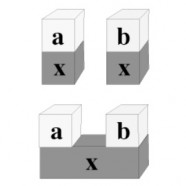In spatial algebra, numbers and variables are represented by physical blocks.  The Image shows the distributive rule.  In space, distribution is simply cutting or joining blocks with the same label.  The symbolic equivalent is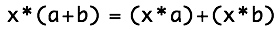Spatial algebra is not a complete algebraic system, since higher powers require more spatial dimensions or very convoluted shapes for  ”blocks”.  Instead, spatial algebra is a learning tool for those first encountering algebra.  The educational approach is to anchor concepts first to experience, and then later to introduce the more abstract symbolic concepts. For novice algebra students, the symbolic form is more prone to errors.  We can say that the structure of symbolic algebra affords, or facilitates, student errors. Conceptual understanding is better supported by iconic systems that can be physically manipulated.

There is, however, a significant conceptual change.  The concepts associated with space are different than those associated with conventional algebraic structures such as groups.  By reintroducing the Additive Principle (that a sum is composed of its parts) into algebra, the concepts of algebra change.  They become much simpler.  A clear example is with the Identity Rule for addition, that there exist a number, 0, that when added to anything does not change that anything.  This rule is written as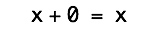The symbol 0 is not part of space, it is part of symbolic notation.  The corresponding concept in space is emptiness, that is , nothing at all.  When an object is put into space, the space around it does not change its identity.  The symbol zero, in fact the concept Zero, is just not relevant to spatial algebra.

This page is a revision of content first published in  W. Bricken (1992) Spatial Representation of Elementary Algebra1992 IEEE Workshop on Visual Languages, Seattle, IEEE Computer Society Press, 56-62. Spatial algebra was also presented at the 1992 meeting of the American Educational Research Association in the context of designing a virtual reality math learning environment for algebra students. The paper Designing Virtual Worlds for Use in Mathematics Education: the Example of Experiential Algebra, written by W. Winn and W. Bricken, was published in Educational Technology, v32(12),12-19.

# Spatial Representation of Elementary Algebra

### Abstract

Our understanding of a concept is tightly connected to the way we represent that concept.  Traditionally, mathematics is presented textually.  As a consequence novice errors (in elementary algebra for example) are due as much to misunderstanding the nature of textual symbols as they are to miscomprehension of the mathematical concepts represented by the symbols.  This page outlines a spatial algebra by mapping the structure of commutative groups in symbolic algebra onto the structure of space in physical reality.  We interact with spatial representations through natural behavior in an inclusive environment.  When the environment enforces the rules of algebra, the spatial representation affords experiential learning and permits algebraic proof through direct manipulation.  Thus the concept structure of spatial algebra is both simpler and more natural and intuitive than the concept structure of conventional symbolic algebra.

## Introduction

How we think about mathematical concepts is influenced by how those concepts are represented.  Syntax and semantics (representation and concept) are tightly connected.  The conventional representation of binary addition is one-dimensional.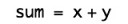There are two locations for what is being added, one on either side of the textual operator, +.   But common addition is not restricted to only two objects.  We could, for example, have memorized the sums of all three digit combinations, so that addition takes three numbers instead of two.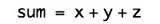Our addition tables would then contain 1000 rather than 100 addition facts.  This would be very inconvenient, but in principle, addition is not binary.  Binary sums are a choice of convenience rather than a conceptual necessity.  In general, how we represent numbers is a matter of convenience, but the convenience of the learner has been forgotten in math education.  Math classrooms teach symbolic notation exclusively, and thus generate confusion rather than understanding for the vast majority of students.  For learning mathematics (and for doing mathematics) it is often more convenient to call upon visual interaction and natural behavior than it is to conduct symbolic substitutions devoid of meaning.  From a spatial perspective, many things can be put together (i.e. added) at the same time.  The number of things being put together into the same space does not matter, it can two or three or many.  One of the objectives of spatial algebra is to identify the natural, intuitive concepts necessary to do algebra, and to contrast these concepts with the concepts introduced by the dominant group theoretic abstract algebra taught in today’s elementary schools.

Spatial algebra uses the three dimensions of natural space to express algebraic concepts.  A higher dimension of representation greatly simplifies the visualization and the application of algebraic ideas.   Algebraic transformation and the process of proof are achieved through direct manipulation of the three-dimensional physical representation of the algebra problem.  For classrooms and webpages dominated by text and illustration rather than physical objects, a compromise is to draw algebraic forms.  These virtual forms can be manipulated as-if real, permitting at least a limited degree of physical interaction with the conceptual forms.

The difficulties children have when they begin to learn algebra are well documented     .  Spatial algebra addresses common errors made by novice algebra students by permitting experiential interaction with virtual forms.  Spatial representations enhance understanding .  Concrete manipulation is known to be an effective teaching technique   .  But the most important idea is that symbolic algebra is too complicated, too unnatural, too inhumane.

Virtual reality is a computer generated, multi-dimensional, inclusive environment which can be accepted by a participant as cognitively valid .  The programmability of virtual forms allows a curriculum designer to embed pedagogical strategies into the behavior of virtual objects that represent mathematical structures .  With virtual forms, the axioms of algebra can be, so to speak, built into the behavior of the world.

The visual programming community has developed taxonomies of visual approaches .  The experiential approach to mathematical formalism presented here is sufficiently unique not to fit into existing taxonomies of visual languages.  The spatial techniques in this paper are general and have been applied to several formal systems, including elementary logic and integer arithmetic  .

Update 2012:  The Algebra Touch iPad/iPhone application from Regular Berry Software nicely illustrates the idea that the symbols of algebra themselves can enforce the rules of algebra.  But twenty years later, the problem of unintuitive symbol manipulations has taken a new twist.  The Wolfram Algebra Course Assistant iPad/iPhone application from Wolfram Alpha uses Mathematica to perform all the algebraic manipulations, freeing the student to focus on using math rather than doing math.  That is, from a modern perspective, we can now leave all computing to the computers that we built for precisely that purpose.  Having students learn algebraic manipulations in the twenty-first century is analogous to asking them to ride a horse to school rather than taking the school bus.

## Mapping Algebra into 3D Space

The aspects of three-dimensional space that are used for the representation of algebraic concepts include:

•  empty space (the void),

•  partitions between spaces (boundaries, objects),

•  labeled objects that share a space, and

•  labeled objects that share a boundary (touch one another).

This is sufficient structure for the expression of elementary algebra.  One possible map from algebraic tokens to physical spaces is: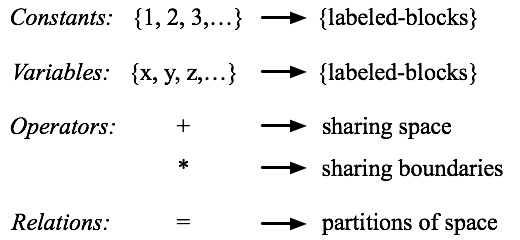Examples of a spatial representation of this map follow.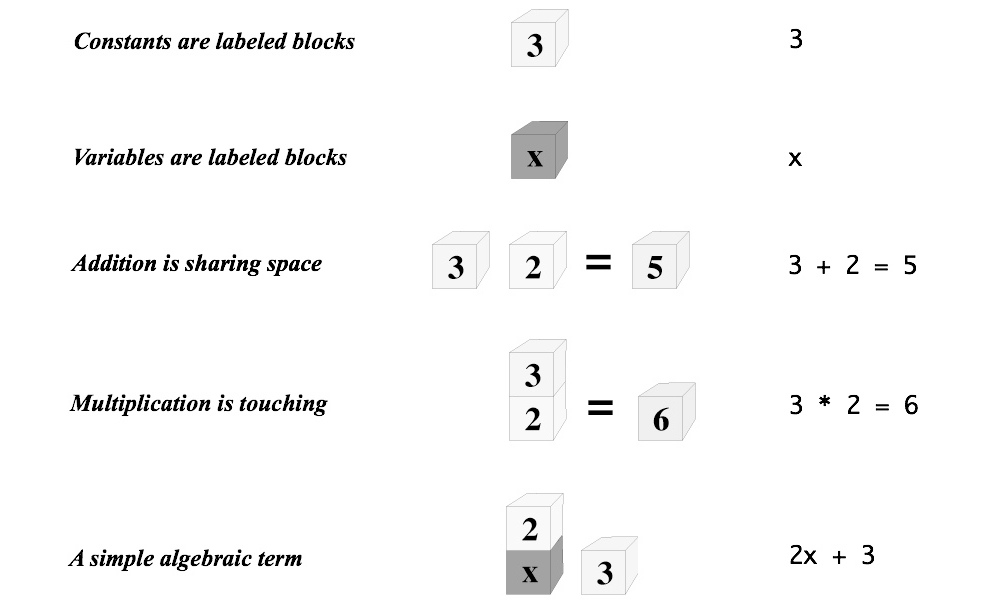The gravitational orientation of the typography (top to bottom of page) in the above examples is not an aspect of spatial algebra, although gravitational metaphors are useful for the representation of sequential concepts such as non-commutativity.  As well, the sequencing implied by stacked blocks is an artifact of typography;  stacks only represent groups of objects touching in space.

## Group Structure of Spatial Forms

Generally, spatial representation can be mapped onto group theory.  A commutative group is a mathematical structure consisting of a set and an operator on elements of that set with the following properties:

•  The set is closed under the operation.

•  The operation is associative and commutative.

•  There is an identity element.

•  Every element has an inverse.

The integer addition and multiplication operators taught in elementary school belong to the commutative group.  In order to create this map, it is necessary to add concepts to spatial algebra, in effect to make it more complicated.  The additional concepts in effect reduce the freedom provided by three-dimensional space to the constraints of a one-dimensional line, a string of symbols.  The commutative, associative and identity rules of group theory are thus constructs to support symbol-strings rather than constructs to support a naturalistic physical understanding of algebra.

### Commutativity

Spatial representation permits the implicit embedding of commutativity in space.  The commutativity of addition is represented by the absence of linear ordering of blocks in space (visualize the blocks in this example as floating in space rather than in a particular linear order).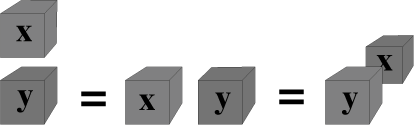Symbolic string equivalent:   x + y = y + x

More accurately, commutativity is not a relevant concept in spatial algebra.  We intuitively recognize objects contained in a three-dimensional space as ordered solely by our personal perspective.  In contrast, typographical objects are necessarily ordered in sequence by the one-dimensional nature of text and by the two-dimensional nature of the page.  In essence, we as readers are not able to move our viewpoint.  This fundamental change in what constitutes mathematical form both simplifies iconic algebra by removing inessential concepts and defines a new kind of mathematics that is not based upon the currently dominant conceptual model that arithmetic must be described within the theory of groups.

The commutativity of multiplication can be seen as the absence of ordering in touching blocks.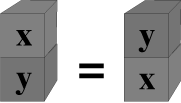Symbolic string equivalent:   x*y = y*x

Again, in space there is no preferential ordering to touching objects: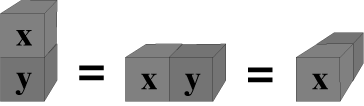Symbolic string equivalent:   x*y = y*x

Abandoning the commutativity of multiplication is more radical than abandoning the commutativity of addition.  It is common experience that the order of multiplication effects the process of multiplication, even though the result is the same.  Multiplying 3 by 2, for example, calls for two copies of 3.  Multiplying 2 by 3 calls for three copies of 2.  This is the case even with concrete manipulables.  The “multiplication-as-touching” model of spatial algebra does not incorporate the process of carrying out the multiplication via physical steps.  Rather the result is achieved immediately and is independent of which number is multiplied by which.  This approach is more abstract than arranging objects in tableaus in space.  Spatial multiplication simply counts the number of elements in the product.

### Associativity

Associativity is also abandoned in the spatial form.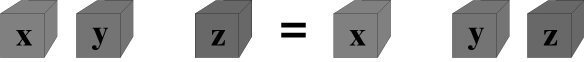Symbolic string equivalent:   (x + y) + z = x + (y + z)

The apparent visual grouping expressed by differences in metric distance between blocks can be taken to mean associativity (for example, add closest objects first), or it can be ignored, permitting the operation assigned to space to address multiple arguments in parallel.  From an intuitive perspective, operations embedded in space apply to any number of objects in that space.  Whatever grouping we use is a matter a choice and convenience.  Associativity comes from two unnecessary structural concepts associated with strings of symbols.  The first is that objects must be combined two-at-a-time.  The closely related second idea is that processing must take place in sequence.  These ideas arose in an era prior to parallel computers and prior to the concurrent display multiple objects provided by computer graphics.

Similarly, the associativity of multiplication is not necessary, since touching objects can all touch at the same time.  The visual metaphor of touching is weak in that it does not convey the interpenetration of the objects implied by multiplication.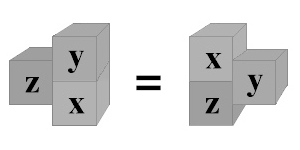Symbolic string equivalent:   (x*y)*z = x*(y*z)

### Distribution

Precedence operations associated with the distributive rule are the most common algebraic error for first year algebra students  , although arithmetic errors with negative numbers and with fractions predominate.  The representation of distribution in spatial algebra is particularly compelling.  Generally, the distributive law permits combining blocks with identical labels into a single block with that label.  Conversely (read right to left), distribution permits splitting a single block that touches separate piles into separate but identical blocks touching each pile: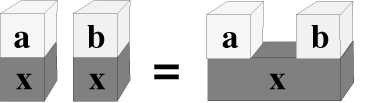Symbolic string equivalent:   a*x + b*x = (a + b)*x

Blocks with identical labels are both singular and arbitrarily subdividable in space.  This ability to arbitrarily divide and combine blocks with a common name is the same as the ability to arbitrarily create duplicate labels in a textual representation.   Any potential ambiguity between replication due to distribution and replication intended as addition is avoided by the effect of context on interpretation.  Distributive replication requires the context of touching blocks (multiplication).  Additive replication requires the context of non-touching piles.

### Identities

Zero is the identity element for addition.  The identity in the spatial metaphor is the void;  identities are equivalent to empty space.  The additive identity requires a “fabrication”, a zero block that is equivalent to no block at all.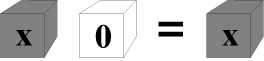Symbolic string equivalent:   x + 0 = x

That is, zero disappears in space: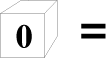Symbolic string equivalent:   0 =

The multiplicative identity is the 1 block.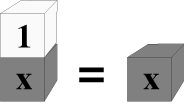Symbolic string equivalent:   1*x = x

The 1 block disappears only in the context of an existing pile.  A zero in a pile makes the entire pile disappear: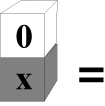Symbolic string equivalent:   0*x = 0

The inverse of a positive number is a negative number.  Negative numbers are the most difficult aspect of arithmetic for elementary students. One way to directly represent inversion is to create an inverter block.  Another way is to create an inversion space; for example using “under-the-table” for inverses.  Inverses can be represented in many ways: as inverters, as colors, as orientations, as different spaces, as binary switches, as dividing planes, as inside-out objects.  In this version of spatial algebra, piles are inverted by the inclusion of a special inverter block.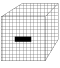Symbolic string equivalent:   (–)

Since a negative number can be seen as being multiplied by -1, the inverter block is expressed as touching (multiplying) the pile that is inverted.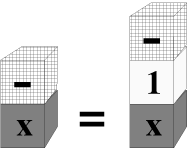Symbolic string equivalent:   –x = (–1)*x

The inverter block expresses subtraction as the addition of inverses.  This modern algebraic perspective permits the concept of subtraction to be omitted from algebraic form.  x – x  is written and conceptualized as  x + (–x).

The additive inverse shows that canceling blocks are simply removed, they are absorbed into the void.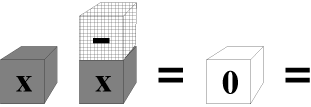Symbolic string equivalent:  x + (-x) = 0 =

## Calculus of Signs

The use of the inverter block for negative numbers introduces a calculus of signs into the algebra of integers.  A sign calculus requires the explicit introduction of the positive block.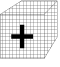Symbolic string equivalent:   (+)

The positive block is the inverse of the inverter block.  It introduces the concept of polarity and the act of cancellation.  Numbers without signs are usually assumed to be positive.  Making signs explicit removes this assumption.  The following rules of sign calculus assume each sign has a unit value associated with it.

For additive cancellation in space, positive and negative blocks sharing a space cancel into nothing.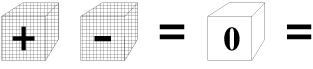Symbolic string equivalent:   (+) + (–) = 0 =

For cardinality in space, replicate positive of negative blocks are assumed to to have the multiplicative identity, 1, attached, so that the units can be added into a sum.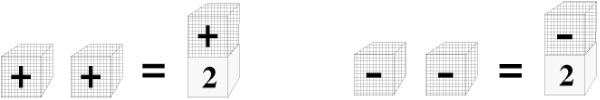Symbolic string equivalent:   (+) + (+) = 2*(+)   and    (–) + (–) = 2*(–)

For multiplicative cancellation in piles, pairs of touching positive blocks or touching negative blocks convert to a single positive block, which by convention of the multiplicative inverse, 1, can then be deleted.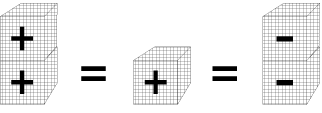Symbolic string equivalent:   (+)*(+) = (+) = (–)*(–)

When different signed blocks are in the same pile, negative blocks dominate positive blocks.  This can again be seen as the convention of not including positive blocks in a pile.  That is, these rules work intuitively in piles by simply deleting all positive blocks (and deleting pairs of negative blocks).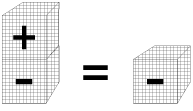Symbolic string equivalent:   (+)*(–) = (–)

The following example illustrates an inverter sign distributed across all objects in a space: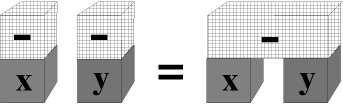Symbolic string equivalent:  (–x) + (–y) = –(x + y)

## Multiplicative Inverse

Finally, division is the multiplicative inverse.  Again, there are many possible ways to represent an inverse in a spatial representation.  Since the traditional notation for fractions is primarily two-dimensional, it already has many spatial aspects.  The division line that separates numerator from denominator could be carried over to the spatial representation as a plane dividing a pile into two parts.  Here however, the multiplicative inverse is represented by inverse shading of the block label: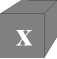Symbolic string equivalent:   1/x

The multiplicative inverse converts a pair of inverse blocks to a single unit.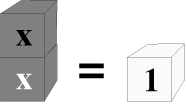Symbolic string equivalent:   x * 1/x = 1

One weakness with the choice to represent a reciprocal as differently shaded labels is that composition of reciprocals — for example 1/(1/x) — is not visually defined.  Choice of representation necessarily effects pedagogy.  It is an empirical question as to which representations more efficiently facilitate learning.

Fractions are the second most difficult area for students of arithmetic.  A typical problem using fractions requires the application of the distributive rule.  Below, a block and its inverse have been inserted into each stack.  The particular block is determined by the inverse block in the other stack.  In this abstract approach, the issue of lowest common denominators does not come up.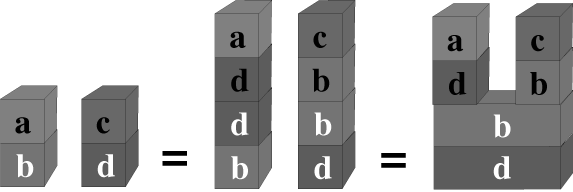Symbolic string equivalent:  a/b + c/d = (a*d + b*c)/(b*d)

## Factoring

Factoring polynomial expressions is equivalent to multiple applications of distribution.  For instance: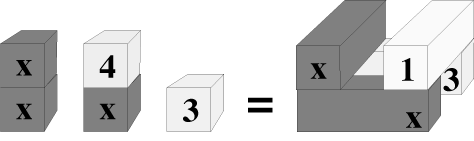Symbolic string equivalent:   x2 + 4x + 3 = (x + 1)*(x + 3)

One advantage of the spatial representation on the right-hand-side of this equation is that both the factored and the polynomial forms are visible concurrently.  Looking from the side, we see two completely touching spaces that represent the factored form.  Looking down from the top, we see four piles that represent the polynomial form.  Here, the factored form is converted to the polynomial by slicing each addition space through the middle.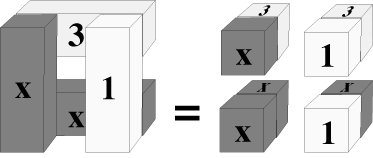Symbolic string equivalent:  (x + 1)*(x + 3) = x2 + 1*x + 3*x + 1*3

## Caveats

Experiential mathematics is quite new as a formalism.  The idea of mapping semantics onto the void first appeared in a mathematical text that is widely acknowledged as impenetrable .  Spatial algebra is an interpretation of the abstract mathematics developed by Spencer-Brown in Laws of Form and by Louis Kauffman at the University of Chicago .

The representational details of the spatial algebra presented here are, like any choice of syntax, somewhat arbitrary.  The text lists many options, for example, for the representation of inverses.  This representational freedom can be constrained by empirical studies intended to determine which particular representations are more effective for task performance.  There is no reason to believe that effective representations are generic.  More probably, different individuals will prefer and understand different representations in the context of different tasks.  Still, the research to determine which representations are effective has yet to be conducted.  In fact, demonstrating that spatial algebra actually improves performance in high school algebra remains as future research.

Significant components of a complete spatial mathematics have not been included on this page.  In particular, a compelling representation for exponentiation is missing.  Spatial arithmetic has been assumed.  A technical refinement is needed for the calculus of signs, to either remove cardinality completely from signs, or embed it deeper, expressing as –1.  We also need to consider representation of functions such as the logarithm and sine.  Spatial solutions to these shortfalls exist, but a completely integrated spatial mathematics is not yet formulated.

The weakest aspect of the proposed spatial  algebra is the representation of three or more multiplied objects, w*x*y*z for example.  This form can be represented by either completely interpenetrating blocks or by “blocks” with complex shapes that twist around to touch all other blocks.  This problem gets particularly difficult for multiplying several factored expressions, for example:  (x + 1)*(x + 2)*(x + 3).

In general, the cubic blocks presented in this paper are misleading, since they imply a Cartesian coordinate system.  In fact, the spatial representation proposed here has no associated metric (or rather, the metric is irrelevant to the mathematical formalism).  The treatment of space might  be improved by explicitly including a representation for the table which blocks can be imagined to rest upon.

Update 2012:  The question of empirical evaluation of spatial algebra for improving math education is subtle.  Spatial algebra teaches a different set of concepts than does symbolic group theory.   If success in school means understanding the manipulation of symbols based on abstract rules, then spatial algebra may even reduce competence.  The problem, however, is with the criteria for success, not with student learning.  Schools have a great difficulty updating concepts and approaches that have been obsoleted by advancing culture and technology.  Manipulation of symbols itself is an anachronism, so that establishing this skill as the criterion for success in high school algebra is plainly dysfunctional.  Many modern improvements in math education speak of conceptual learning rather than memorization of algorithms.  However, still today 90% of math classrooms are mired in what is rapidly becoming ancient history.  Symbolic algebra lacking computer support for computation is as dead as learning ancient Greek and as intellectually misguided as studying the phlogistine theory of combustion.

## Conclusion

Spatial representation provides a map to a wide range of new visual languages.  The examples in this paper are expressed in a language of labeled blocks.  The spatial rules, however, map just as easily onto people in a room, toys in a box, salmon in streams, and bricks in a wall.  The techniques of spatial algebra and the display capabilities of computer systems have coevolved.  Spatial algebra is proposed as an experimental approach for exploring the representation-dependent aspects of novice algebra errors.  Computer graphic display systems are a straightforward way to present spatial algebra as an experiential mathematical system.

Here’s an example of using spatial algebra to simplify a deeply nested algebraic expression.  The example in symbolic notation is: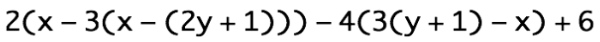And here is a conventional solution by successively multiplying out the nested terms and collecting like terms when possible.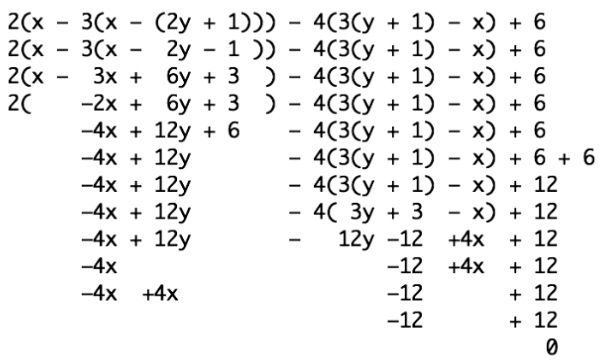Now here’s the same expression simplified using spatial algebra.  First the expression is represented as a stack of blocks.  For this example, the blocks are two-dimensional.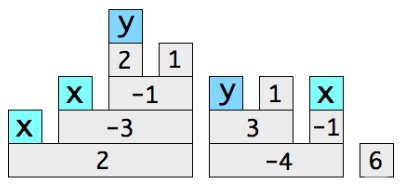The first step is to cut through all the shared blocks, in effect applying distribution to the entire expression all at the same time.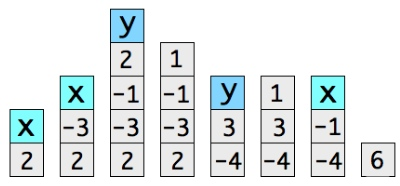Next, as done in the algebraic simplification, we will assume knowledge of the number facts.  Rather than applying them one-by-one, all the multiplications occur at the same time.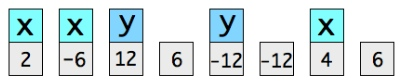Now all that is left is to join like terms.  All of the stacks are rearranged here due to the two-dimensional representation on the page.  In space, the rearrangement activity is more like gathering than putting stacks next to one another.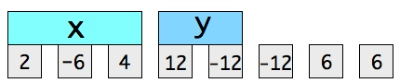Again, number facts are applied to each different term, all at the same time.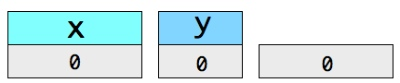In spatial notation, what remains is zero, which would be erased, leaving nothing at all, i.e. the empty page.

### References

  Berman, B., & Friederwitzer, F. (1989)  Algebra can be elementary … When it’s concrete  Arithmetic Teacher, 36 (8), 21-24.

  Bricken, M. (1991)  Virtual reality learning environments:  potentials and challenges  Computer Graphics Magazine, ACM, 7/91.

  Bricken, W. (1986)  A simple space  Proceedings of the Sign and Space Conference, University of California at Santa Cruz.  Also as HITL Technical Report R-86-3 , University of Washington, 1989.

  Bricken, W. (1987)  Analyzing errors in elementary mathematics  Doctoral dissertation, School of Education, Stanford University.

  Bricken, W. (1989)  An introduction to boundary logic with the Losp deductive engine  Future Computing Systems 2-4.

  Bricken, W. (1992)  Virtual reality:  directions of growth  Proceedings, Imagina’92, Centre National de la Cinematographie, Monte-Carlo.

  Gerace, W.J., & Mestre, J.P. (1982)  The learning of algebra by 9th. graders: Research findings relevant to teacher training & classroom practice  Final Report, National Institutes of Health, Washington DC, (Contract # 400-81-0027).

  Greeno, J. (1985)  Investigations of a cognitive skill  Technical Report, Pittsburgh: University of Pittsburgh Learning and Development Center.

  Kaput, J.J. (1978)  Mathematics and learning: roots of epistemological status  In J. Lochhead & J. Clements (Eds.), Cognitive process instruction  Philadelphia, PA: Franklin Institute Press.

  Kauffman, L. (1980)  Form dynamics  Journal of Social and Biological Structures  3, 171-206.

  Larkin, J.H., & Simon, H.A. (1987)  Why a diagram is (sometimes) worth ten thousand words  Cognitive Science, 11, 65-99.

  National Assessment of Educational Progress (1981)  Results from the second mathematics assessment  National Council of Teachers of Mathematics, Reston, Va.

  Shu, N. C. (1988)  Visual programming  Van Nostrand Reinhold, New York.

  Shumway, R.J. (1989)  Solving equations today  School Science and Mathematics, 89, 208-219.

  Sowell, E.J. (1989)  Effects of manipulative material in mathematics instruction  Journal of Research in Mathematics Education, 20, 498-505.

  Spencer-Brown, G. (1972)  Laws of form  Julian Press, New York.

  Thwaites, G.N. (1982)  Why do children find algebra difficult?  Mathematics in school, 11(4), 16-19.

  Winn, W. & Bricken, W. (1992)  Designing virtual worlds for use in mathematics education  Proceedings of AERA, 1992.